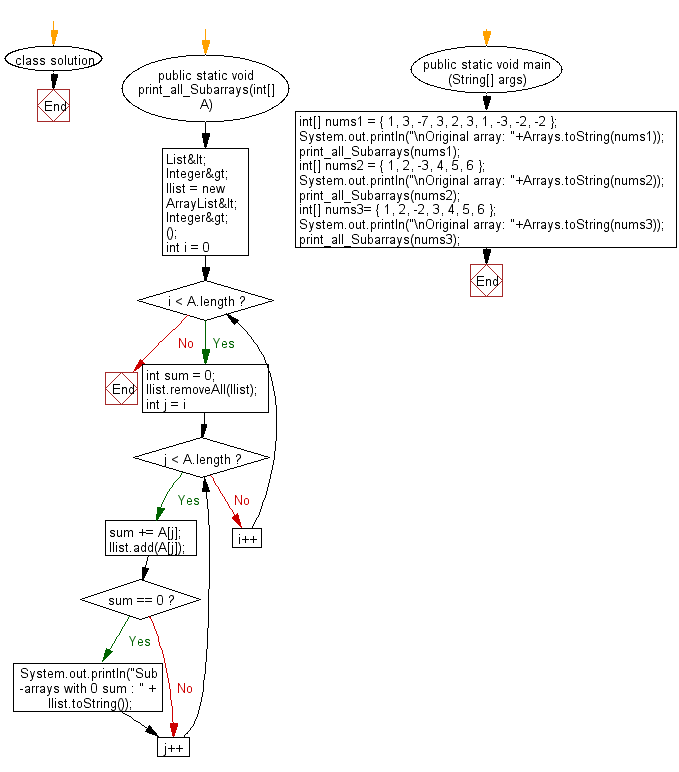﻿ Java: Print all sub-arrays with 0 sum present in an array# Java Array Exercises: Print all sub-arrays with 0 sum present in a given array of integers

## Java Array: Exercise-55 with Solution

Write a Java program to print all sub-arrays with 0 sum present in a given array of integers.

Example:
Input :
nums1 = { 1, 3, -7, 3, 2, 3, 1, -3, -2, -2 }
nums2 = { 1, 2, -3, 4, 5, 6 }
nums3= { 1, 2, -2, 3, 4, 5, 6 }
Output:
Sub-arrays with 0 sum : [1, 3, -7, 3]
Sub-arrays with 0 sum : [3, -7, 3, 2, 3, 1, -3, -2]
Sub-arrays with 0 sum : [1, 2, -3]
Sub-arrays with 0 sum : [2, -2]

Sample Solution:

Java Code:

``````import java.util.*;
import java.lang.*;
class solution
{
public static void print_all_Subarrays(int[] A)
{

List<Integer> llist = new ArrayList<Integer>();
for (int i = 0; i < A.length; i++)
{
int sum = 0;
llist.removeAll(llist);
for (int j = i; j < A.length; j++)
{
sum += A[j];
if (sum == 0) {
System.out.println("Sub-arrays with 0 sum : " + llist.toString());

}
}
}
}

public static void main (String[] args)
{
int[] nums1 = { 1, 3, -7, 3, 2, 3, 1, -3, -2, -2 };
System.out.println("\nOriginal array: "+Arrays.toString(nums1));
print_all_Subarrays(nums1);

int[] nums2 = { 1, 2, -3, 4, 5, 6 };
System.out.println("\nOriginal array: "+Arrays.toString(nums2));
print_all_Subarrays(nums2);

int[] nums3= { 1, 2, -2, 3, 4, 5, 6 };
System.out.println("\nOriginal array: "+Arrays.toString(nums3));
print_all_Subarrays(nums3);
}
}
```
```

Sample Output:

```
Original array: [1, 3, -7, 3, 2, 3, 1, -3, -2, -2]
Sub-arrays with 0 sum : [1, 3, -7, 3]
Sub-arrays with 0 sum : [3, -7, 3, 2, 3, 1, -3, -2]

Original array: [1, 2, -3, 4, 5, 6]
Sub-arrays with 0 sum : [1, 2, -3]

Original array: [1, 2, -2, 3, 4, 5, 6]
Sub-arrays with 0 sum : [2, -2]
```

Flowchart:Java Code Editor:

Improve this sample solution and post your code through Disqus

What is the difficulty level of this exercise?

Test your Programming skills with w3resource's quiz.

﻿

## Java: Tips of the Day

countOccurrences

Counts the occurrences of a value in an array.

Use Arrays.stream().filter().count() to count total number of values that equals the specified value.

```public static long countOccurrences(int[] numbers, int value) {
return Arrays.stream(numbers)
.filter(number -> number == value)
.count();
}
```

Ref: https://bit.ly/3kCAgLb